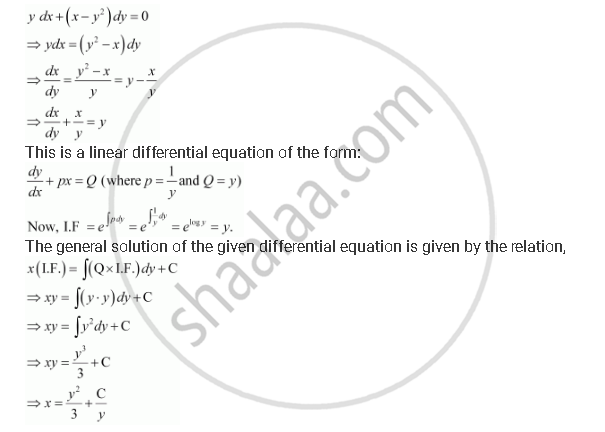Share

# For the Differential Equations Find the General Solution: Y Dx + (X – Y2) Dy = 0 - CBSE (Science) Class 12 - Mathematics

ConceptMethods of Solving First Order, First Degree Differential Equations Linear Differential Equations

#### Question

For the differential equations find the general solution:

y dx + (x – y2) dy = 0

#### SolutionIs there an error in this question or solution?

#### APPEARS IN

NCERT Solution for Mathematics Textbook for Class 12 (2018 to Current)
Chapter 9: Differential Equations
Q: 11 | Page no. 414

#### Video TutorialsVIEW ALL 

Solution For the Differential Equations Find the General Solution: Y Dx + (X – Y2) Dy = 0 Concept: Methods of Solving First Order, First Degree Differential Equations - Linear Differential Equations.
S